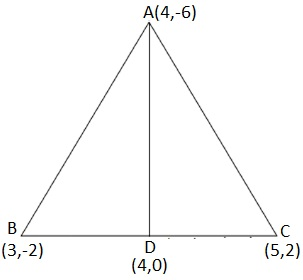Newbie

# You have studied in Class IX that a median of a triangle divides it into two triangles of equal areas. Verify this result for ΔABC whose vertices are A (4, – 6), B (3, – 2) and C (5, 2). Q.5

• 0

Google please help me to find the best solution of class 10th exercise 7.3 coordinate geometry. You have studied in Class IX that a median of a triangle divides it into two triangles of equal areas. Verify this result for ΔABC whose vertices are A (4, – 6), B (3, – 2) and C (5, 2).

Share

1. Let the vertices of the triangle be A (4, -6), B (3, -2), and C (5, 2).Let D be the mid-point of side BC of ΔABC. Therefore, AD is the median in ΔABC.

Coordinates of point D = Midpoint of BC = ((3+5)/2, (-2+2)/2) = (4, 0)

Formula, to find Area of a triangle = 1/2 × [x1(y2 – y3) + x2(y3 – y1) + x3(y1 – y2)]

Now, Area of ΔABD = 1/2 [(4) {(-2) – (0)} + 3{(0) – (-6)} + (4) {(-6) – (-2)}]

= 1/2 (-8 + 18 – 16)

= -3 square units

However, area cannot be negative. Therefore, area of ΔABD is 3 square units.

Area of ΔACD = 1/2 [(4) {0 – (2)} + 4{(2) – (-6)} + (5) {(-6) – (0)}]

= 1/2 (-8 + 32 – 30) = -3 square units

However, area cannot be negative. Therefore, the area of ΔACD is 3 square units.

The area of both sides is the same. Thus, median AD has divided ΔABC in two triangles of equal areas.

• 0
• Share